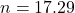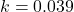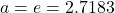## The whooping crane (Grus americana) is the tallest bird native to North America. It almost went extinct in the 1900s; in 1938, there were on

Question

The whooping crane (Grus americana) is the tallest bird native to North America. It almost went extinct in the 1900s; in 1938, there were only 18 whooping cranes left in the wild. Since then, its population has increased. The number of whooping cranes (N) is related to the number of decades since 1938 (t) by the following expression: ln(N) = 2.85 + 0.039t.
The exponential relationship can be written as N = nakt, where n, a, and k are constants. Determine the value of a.

in progress 0
2 months 2021-07-28T01:06:07+00:00 1 Answers 17 views 0

The value  is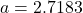Explanation:

From the question we are told that

The  relationship between the number of whooping cranes and the number of decades is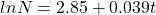The  exponential relationship is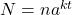Now from the given equation we have that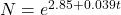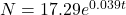So comparing this equation obtained an the given  exponential relationship we have that